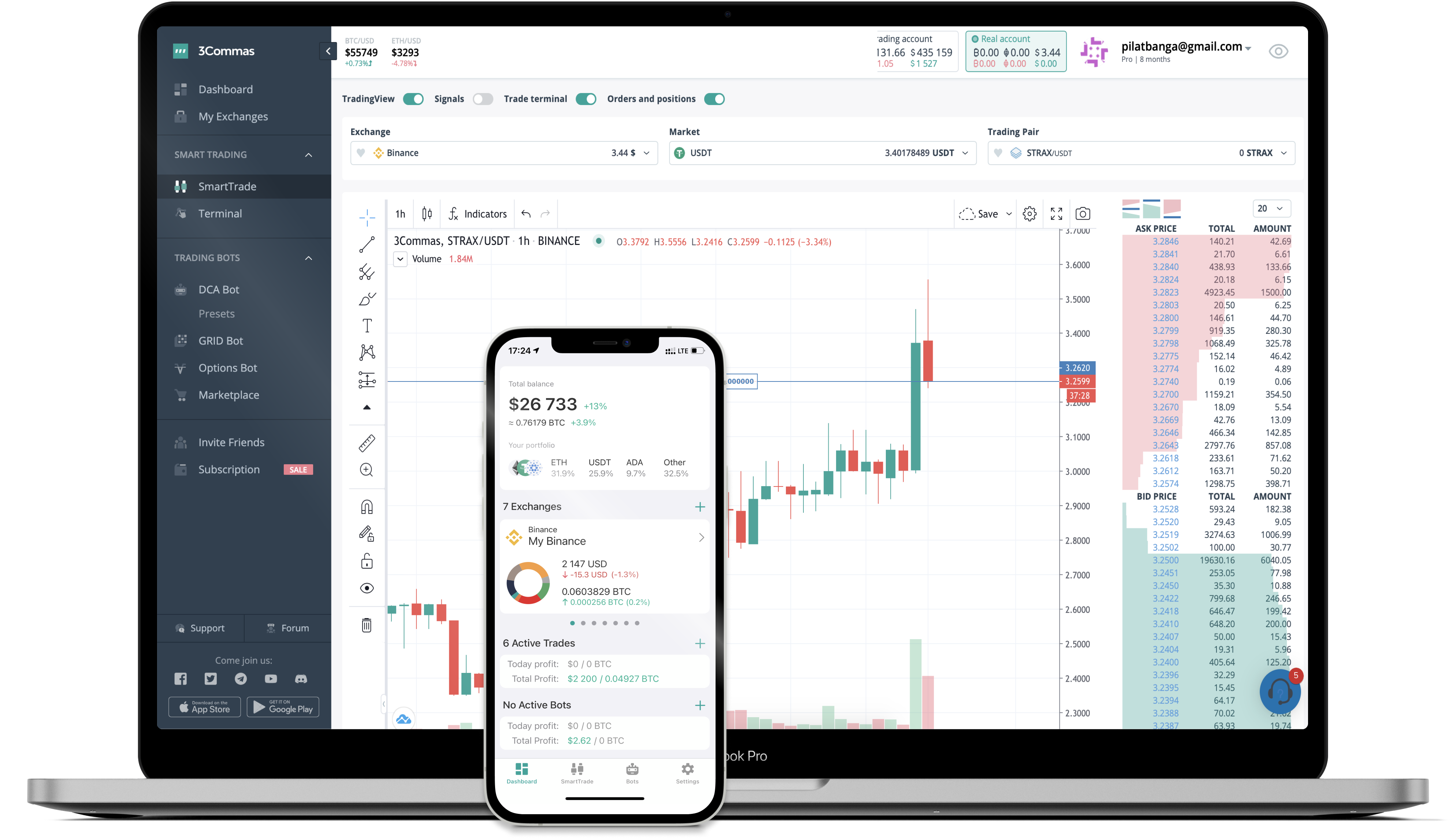# VECT to INR Converter

Vectorium
PriceINR 43,650.747-7.46%
VolumeINR 122,177,460
How it works

The 3Commas currency calculator allows you to convert a currency from Vectorium (VECT) to Indian Rupee (INR) in just a few clicks at live exchange rates.

Simply enter the amount of Vectorium you wish to convert to INR and the conversion amount automatically populates. You can also use our Prices Calculator Table to calculate how much your currency is worth in other denominations, i.e. .1 VECT, .5 VECT, 1 VECT, 5 VECT, or even 10 VECT.

## VECT to INR Exchange Calculator

The chart displays Vectorium price changes in INR.

The ultimate tools for crypto traders to maximize trading profits while minimizing risk and loss.## Latest VECT to INR price calculator

Vectorium market price is updated every three minutes and is automatically displayed in INR. Below are the most popular denominations to convert to INR.

Vectorium
Indian Rupee
0.01
VECT
436.51
INR
0.1
VECT
4365.07
INR
1
VECT
43650.75
INR
2
VECT
87301.49
INR
3
VECT
130952.24
INR
5
VECT
218253.73
INR
10
VECT
436507.47
INR
25
VECT
1091268.67
INR
50
VECT
2182537.35
INR
100
VECT
4365074.70
INR
250
VECT
10912686.74
INR
500
VECT
21825373.48
INR
1000
VECT
43650746.96
INR
2500
VECT
109126867.41
INR
Indian Rupee
Vectorium
0.01
INR
0.00000023
VECT
0.1
INR
0.00000229
VECT
1
INR
0.00002291
VECT
2
INR
0.00004582
VECT
3
INR
0.00006873
VECT
5
INR
0.00011455
VECT
10
INR
0.00022909
VECT
25
INR
0.00057273
VECT
50
INR
0.00114546
VECT
100
INR
0.00229091
VECT
250
INR
0.00572728
VECT
500
INR
0.01145456
VECT
1000
INR
0.02290912
VECT
2500
INR
0.05727279
VECT

## FAQ

### How much is 1 Vectorium in Indian Rupee?

Vectorium price in INR is constantly changing.

At this moment, 1 Vectorium equals 43650.746964657534 INR

### How do I calculate the conversion from VECT to INR?

The 3Commas Vectorium Calculator allows you to easily calculate the conversion price of VECT to INR by simply entering the amount of Vectorium in the corresponding field and will automatically convert the value in Indian Rupee (INR).

You can also use our Vectorium price table above to check the latest Vectorium price in major fiat and crypto currencies.

### How can I convert Vectorium to INR?

The most common way of converting VECT to INR is by using a Crypto Exchange or a P2P (person-to-person) exchange platform like LocalBitcoins, etc.